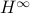# Navid Azizan## A Characterization of Stochastic Mirror Descent Algorithms and their Convergence Properties

N. Azizan R., and B. Hassibi

Proceedings of the IEEE International Conference on Acoustics, Speech and Signal Processing (ICASSP), 2019Stochastic mirror descent (SMD) algorithms have recently garnered a great deal of attention in optimization, signal processing, and machine learning. They are similar to stochastic gradient descent (SGD), in that they perform updates along the negative gradient of an instantaneous (or stochastically chosen) loss function. However, rather than update the parameter (or weight) vector directly, they update it in a ‘‘mirrored’’ domain whose transformation is given by the gradient of a strictly convex differentiable potential function. SMD was originally conceived to take advantage of the underlying geometry of the problem as a way to improve the convergence rate over SGD. In this paper, we study SMD, for linear models and convex loss functions, through the lens ofestimation theory and come up with a minimax interpretation of the SMD algorithm which is the counterpart of the-optimality of the SGD algorithm for linear models and quadratic loss. In doing so, we identify a fundamental conservation law that SMD satisfies and use it to study the convergence properties of the algorithm. For constant step size SMD, when the linear model is over-parameterized, we give a deterministic proof of convergence for SMD and show that from any initial point, it converges to the closest point in the space of all parameter vectors that interpolate the data, where closest is in the sense of the Bregman divergence of the potential function. This property is referred to as implicit regularization: with an appropriate choice of the potential function one can guarantee convergence to the minimizer of any desired convex regularizer. For vanishing step size SMD, and in the standard stochastic optimization setting, we give a direct and elementary proof of convergence for SMD to the ‘‘true’’ parameter vector which avoids ergodic averaging or appealing to stochastic differential equations.